xScattering amplitudeEncyclopedia
In quantum physics, the scattering amplitude is the amplitude of the outgoing spherical wave relative to the incoming plane wave in the stationary-state scattering process. The latter is described by the wavefunction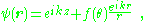where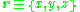is the coordinate vector;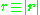;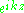is the incoming plane wave with the wave-vector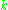along theaxis;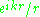is the outgoing spherical wave;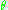is the scattering angle; and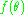is the scattering amplitude. The dimension of the scattering amplitude is length
Length
In geometric measurements, length most commonly refers to the longest dimension of an object.In certain contexts, the term "length" is reserved for a certain dimension of an object along which the length is measured. For example it is possible to cut a length of a wire which is shorter than wire...

.

The differential cross-section
Cross section (physics)
A cross section is the effective area which governs the probability of some scattering or absorption event. Together with particle density and path length, it can be used to predict the total scattering probability via the Beer-Lambert law....

is given as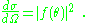In the low-energy regime the scattering amplitude is determined by the scattering length.

## Partial wave expansion

In the partial wave expansion the scattering amplitude is represented as a sum over the partial waves,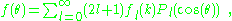where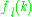is the partial amplitude and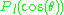is the Legendre polynomial.

The partial amplitude can be expressed via the S-matrix element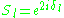and the scattering phase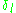as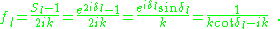## X-rays

The scattering length for X-rays is the Thompson scattering length or classical electron radius
The classical electron radius, also known as the Lorentz radius or the Thomson scattering length, is based on a classical relativistic model of the electron...

,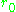.

## Neutrons

The nuclear neutron scattering process involves the coherent neutron scattering length, often described by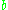.

## Quantum mechanical formalism

A quantum mechanical approach is given by the S matrix
S matrix
In physics, the scattering matrix relates the initial state and the final state of a physical system undergoing a scattering process...

formalism.